# ISEE Middle Level Quantitative : Nets

## Example Questions

### Example Question #257 : Plane Geometry

Which is the greater quantity?

(a) The sidelength of a cube with surface area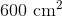(b) The sidelength of a cube with volume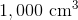(b) is greater

(a) and (b) are equal

(a) is greater

It is impossible to tell from the information given

(a) and (b) are equal

Explanation:

(a) A cube has six faces, each a square. Since the surface area of this cube is, each face has one-sixth this area, or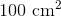; the sidelength is the square root of this, or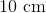.

(b) The volume of a cube is the cube of its sidelength, so we take the cube root of the volume of this cube to get the sidelength: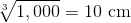The cubes have the same sidelength.

### Example Question #258 : Plane Geometry

Which is the greater quantity?

(a) The volume of a rectangular prism with length 60 centimeters, width 30 centimeters, and height 15 centimeters

(b) The volume of a cube with sidelength 300 millimeters

It is impossible to tell from the information given

(b) is greater

(a) is greater

(a) and (b) are equal

(a) and (b) are equal

Explanation:

(a) The volume of the prism is the product of its length, its width, and its height: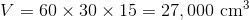(b) The volume of the cube is the cube of its sidelength. We restate 300 millimeters as 30 centimeters, and cube this: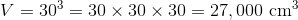The volumes are equal.